# Number unknown

Adela thought the two-digit number, she added it to its ten times and got 407. What number does she think?

Result

a =  37

#### Solution:

a+10a = 407

11a = 407

a = 37

Calculated by our simple equation calculator.

Leave us a comment of example and its solution (i.e. if it is still somewhat unclear...):

Showing 0 comments:Be the first to comment!#### To solve this verbal math problem are needed these knowledge from mathematics:

Do you have a linear equation or system of equations and looking for its solution? Or do you have quadratic equation?

## Next similar examples:

1. NumberDetermine unknown number if you know that difference between five times and triple of number is 42.
2. Unknown number xyzFind the number that its triple is 24. Solve by equation.
3. Seven timesWhich number seven times is just as higher as 27, how much is smaller than 29?
4. Number of songs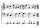Write an expression for the number of songs they need for this show. Evan and Peter have a radio show that has 2 parts. They need 4 fewer than 11 songs in the first part. In the second part, they need 5 fewer than 3 times the number of songs in the first p
5. I thinkI think a number. When I multiply it by five, and after that I subtract 477, I get the same number as if I multiplied it twice. What number do I think?
6. What is missing (1000)What number is to add to get 1000?
7. To thousands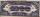Round to thousands following numbers:
8. Roman numeralsWrite numbers written in Roman numerals as decimal.
9. Expression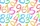If A=2 B=3 evaluate expression A(B+A) and multiply it by A
10. SummandOne of the summands is 145. The second is 10 more. Determine the sum of the summands.
11. Examination 2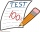In an examination, a teacher awards 2 marks for every correct answer and subtract a mark for every wrong answer. If there are 20 questions and a child scored 25 marks how many correct answer did the child get?
12. Father and son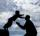Father is 44 years old, his son 16 years. Determine how many years ago was the father five times older than the son.
13. Hotel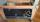The rooms in the mountain hotel are double and triple. Double rooms are 25 and triple are 17 more. How many rooms are there in this hotel?
14. If-then equationIf 5x - 17 = -x + 7, then x =
15. Eq1Solve equation: 4(a-3)=3(2a-5)
16. Six te 2If 3t-7=5t, then 6t=
17. PopsiclesFrancis went to buy ice lollies. If he buy 8 popsicles he missed 4 USD. When he buy 7 popsicles, got back 1 USD. How many USD was a popsicle?